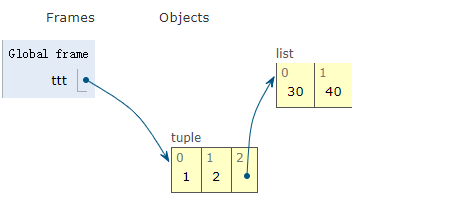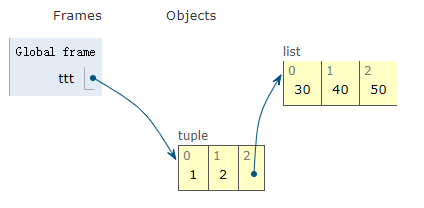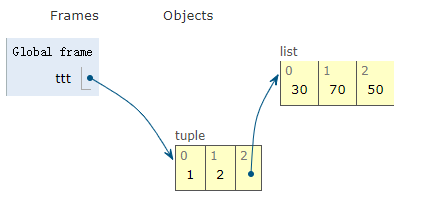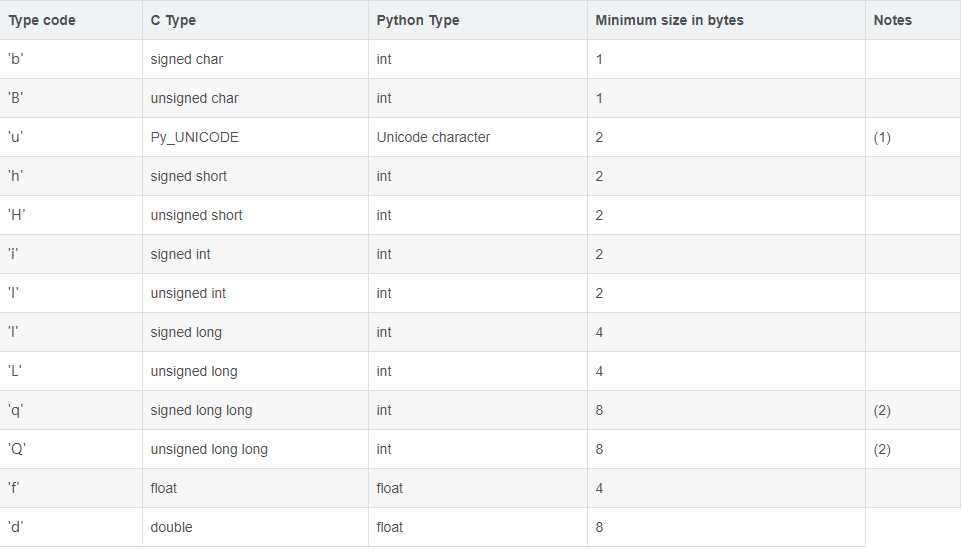# Python数据结构——序列构成的数据之内置序列

hresh 287## 内置序列类型

- 容器序列——容器序列存放的是它们所包含的任意类型的对象的引用；主要包括 list、tuple 和 collections.deque
- 扁平序列——扁平序列里存放的是值而不是引用，换句话说，扁平序列其实是一段连续的内存空间。主要包含 str、bytes、bytearray、memoryview 和 array.array

- 可变序列：list、bytearray、array.array、collections.deque 和 memoryview
- 不可变序列：tuple、str 和 bytes

### 1.首先是文本序列类型——str

s1 = 'hello'
print(s1)
print(id(s1))

s1 = 'world'
print(s1)
print(id(s1))

hello
2187069513944
world
2187069514896


### 2.列表类型——list

classmates = ['Michael', 'Bob', 'Tracy']
print(id(classmates))
print(classmates)

classmates.append('Nike')
print(id(classmates))
print(classmates)

classmates.extend(['Kitty'])
print(id(classmates))
print(classmates)

1594487562824
['Michael', 'Bob', 'Tracy']
1594487562824
['Michael', 'Bob', 'Tracy', 'Nike']
1594487562824
['Michael', 'Bob', 'Tracy', 'Nike', 'Kitty']


### 3.元组类型——tuple

# 在显示只有1个元素的tuple时，也会加一个逗号,，以免你误解成数学计算意义上的括号。
tt = (1,)
print(tt)
# tt = (1)#定义的不是tuple，是1这个数！
# print(tt)
# >>1


ttt = (1,2,[30,40])
ttt.append(50)
ttt = 70### 4.双向列表类型——collections.deque

Deque队列是由栈或者queue队列生成的。Deque 支持线程安全，内存高效添加(append)和弹出(pop)，从两端都可以，两个方向的大概开销都是 O(1) 复杂度。虽然 list 对象也支持类似操作，不过这里优化了定长操作和 pop(0) 和 insert(0, v) 的开销。它们引起 O(n) 内存移动的操作，改变底层数据表达的大小和位置。

from collections import deque

dq = deque(['a','b','c'])
dq.append('x')
dq.appendleft('y')
print(dq)
>>deque(['y', 'a', 'b', 'c', 'x'])


### 5.字节序列—bytes

bytes 数据类型在所有的操作和使用甚至内置方法上和字符串数据类型基本一样，也是不可变的序列对象。

b = b''         # 创建一个空的bytes
b = bytes()      # 创建一个空的bytes
print(b)
b = b'hello'    #  直接指定这个hello是bytes类型
print(b)

#bytes与str相互转换
b = bytes('string',encoding='utf-8')  #利用内置bytes方法，将字符串转换为指定编码的bytes
print(b)
b = 'world'.encode('utf-8')   # 利用字符串的encode方法编码成bytes，默认为utf-8类型
print(b)
# s = b'beijing'.decode('utf-8')
s = b'beijing'.decode()#直接以默认的utf-8编码解码bytes成string
print(s)

b''
b'hello'
b'string'
b'world'
beijing


### 6.字节数组—bytearray

bytearray 是字节组成的有序的可变序列。

ba = bytearray()
print(ba)
ba = bytearray(b'hello Python')
print(ba)

ba = bytearray('hello Python','utf-8')
print(id(ba))
ba.append(21)
print(id(ba))
del ba[5:7]
print(ba)
print(id(ba))

ba = bytearray([94, 91, 101, 125, 111, 35, 120, 101, 115, 101, 200])
print(ba)

bytearray(b'')
bytearray(b'hello Python')
2578379269264
2578379269264
bytearray(b'helloython\x15')
2578379269264
bytearray(b'^[e}o#xese\xc8')


### 7.memoryview 对象

memoryview 对象允许python代码访问支持缓存协议的对象的内部数据而不要 copy。内存通常被理解为简单的字节。注意，不能修改 memoryview 对象的大小。

memoryview 为支持 buffer protocol[1,2] 的对象提供了按字节的内存访问接口，好处是不会有内存拷贝。

• 不使用 memoryview
a = 'aaaaa'
b = a[:2]   # 会产生新的字符串
print(b)

a = bytearray(b'aaaaa')
b = a[:2]   # 会产生新的bytearray
b[:2] = b'bb'   # 对b的改动不影响a
print(b)
print(a)

输出：
aa
bytearray(b'bb')
bytearray(b'aaaaa')

• 使用 memoryview
a = b'aaaaa'
ma = memoryview(a)
print(ma.tobytes())

mb = ma[:2]  # 不会产生新的字符串
print(mb.tobytes())

a = bytearray(b'aaaaa')
ma = memoryview(a)

mb = ma[:2] # 不会会产生新的bytearray
mb[:2] = b'bb'   # 对mb的改动就是对ma的改动
print(mb.tobytes())
print(ma.tobytes())

输出：
True
b'aaaaa'
b'aa'
False
b'bb'
b'bbaaa'


### 8.array.arrayNote: 这里只是规定了对应的最小字节，而不是真实占用的内存字节数！！！如lz使用的 64 位机测试的'i'对应的字节数为 4 而不是 2（32 位机才是 2 吧可能）！

import array

a = array.array('i')
print(a.__sizeof__())
#64
a = array.array('i',)
print(a.__sizeof__())
#68
print(a.itemsize)
#4


a = array.array('i')
a.append(3)
a.extend(range(3))
print(a)
a.pop() #删除最后一个
print(a)


import random

a = array.array('i', [random.randint(1, 100) for _ in range(10)])
print(a)

# 从Python3.4开始，数组（array）类型不再支持诸如list.sort()这种排序方法。
a = array.array(a.typecode, sorted(a))
print(a)
print(a.tolist())

import bisect

i = 50

bisect.insort(a,i)
print(a)


array('i', [95, 46, 62, 85, 27, 36, 12, 24, 96, 71])
array('i', [12, 24, 27, 36, 46, 62, 71, 85, 95, 96])
[12, 24, 27, 36, 46, 62, 71, 85, 95, 96]
array('i', [12, 24, 27, 36, 46, 50, 62, 71, 85, 95, 96])